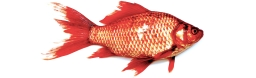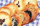# Yellow tangs

In an aquarium 2/5 of the fish are surgeonfish.  Of these 3/4 are yellow tangs.  What fraction of all fish in the aquarium are yellow tangs?

x =  0.3

### Step-by-step explanation:Did you find an error or inaccuracy? Feel free to write us. Thank you!Tips to related online calculators
Need help to calculate sum, simplify or multiply fractions? Try our fraction calculator.

## Related math problems and questions:

• Fractions and mixed numerals(a) Convert the following mixed numbers to improper fractions. i. 3 5/8 ii. 7 7/6 (b) Convert the following improper fraction to a mixed number. i. 13/4 ii. 78/5 (c) Simplify these fractions to their lowest terms. i. 36/42 ii. 27/45 2. evaluate the follow3 3/4 + 2 3/5 + 5 1/2 Show your solution.
• Fraction of a NumberIf 1/2 of 1/3 of 1/4 of 1/5 of a number is 2.5. what is the number?
• Unknown number4/5 of a number is 276. what is 2/3 of the same number?
• The buildingThere are 45 people living in building, out of these 3/5 are men,2/5 are women . find the number of men and women in the building.
• Equivalent fractions 2Write the equivalent multiplication expression. 2 1/6÷3/4
• CompareCompare fractions (34)/(3) and (12)/(4). Which fraction of the lower?
• 5 2/55 2/5 hours a week  mathematics,  3 3/4 hours a week   Natural sciences, 4 3/8 hours a week  Technology . how many hours does  he spend on social sciences if he spend 17 1/2 hours a week for the four subject?
• A land 2A land owner owns two parcels of land measuring 4 2/5 hectares and 6 1/5 hectares. He sells 3/4 of the total land. How many hectares of the land are left?
• Numbers divisionWith what number should be divided mixed number 2 3/4 to get 11/12?In a cookie jar, 1/4 of the cookies are chocolate chip and 1/2 of the rest are peanut butter. What fraction of all the cookies are peanut butter?Which one of the following fraction after reducing in simplest form is not equal to 3/2? a) 15/20 b) 12/8 c) 27/18 d) 6/4Alexandra made a rectangular quilt the measured 3 1/4. 2 3/4 feet in width. What is the area of the quilt in square feet? Write an equation to solve.Write the fraction 3/22 as a decimal.Simplify mixed numerals expression: 8 1/4- 3 2/5 - (2 1/3 - 1/4) Show your solution.Solve following simple equation with fractions: -5/6(8+5b) = 75 + 5/3bWhat is the quotient of 3/10 divided by 2/4 as a fraction?